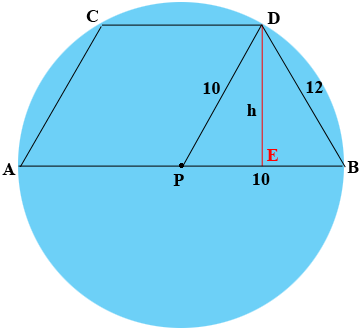SEARCH HOMEMath Central Quandaries & QueriesQuestion from Kameron: I have been given a challenge problem that states that Diameter AB is drawn in a circle if 10 inch. Chords AC and BD are drawn so that each is of length 12inch and ACDB is a trapezoid. Find the height, in inches, of the trapezoidHi Kameron,

Can you check the wording of the question? I don't understand "if 10 inch". What does the ten inches measure?

Penny

Kameron

Hi Kameron,

I drew a diagram (not to scale). $P$ is the center of the circle and $DE$ is perpendicular to PB.$$h = |DE|$is the height of the trapezoid and it's also the height of the triangle$DPB$with base$PB.$The area$A$of the triangle$DPB$is given by $A = \frac12 |PB| \times h = 5 h.$ If you could find the area of the triangle$DPB$a different way then you would have$A = 5 h$and you could solve for$h.$Can you find the area of the triangle$DPB?\$

PennyMath Central is supported by the University of Regina and The Pacific Institute for the Mathematical Sciences.